UGC NET CS 2013 Sep-paper-2

 Question 1
A file is downloaded in a home computer using a 56 kbps MODEM connected to an Internet Service Provider. If the download of file completes in 2 minutes, what is the maximum size of data downloaded ?
 A 112 Mbits B 6.72 Mbits C 67.20 Mbits D 672 Mbits
Question 1 Explanation:
Given data,
-- A file downloaded per second=56 kbps
-- If the file completes in 2 min then maximum size=?
Step-1: Convert minutes into seconds
Given 2 min= 2*60 sec
= 120 sec
Step-2: Downloaded speed per second= 56 kbps
= 56 kbps * 120 sec
= (56 * 1024 *120) / 1024
= 6720 Kbps
= 6.72 Mbits
 Question 2
In ______ CSMA protocol, after the station finds the line idle, it sends or refrains from sending based on the outcome of a random number generator.
 A Non-persistent B 0-persistent C 1-persistent D p-persistent
Computer-Networks       CSMA
Question 2 Explanation:
In p-persistent CSMA protocol, after the station finds the line idle, it sends or refrains from sending based on the outcome of a random number generator.
1-persistent: 1-persistent CSMA is an aggressive transmission algorithm. When the transmitting node is ready to transmit, it senses the transmission medium for idle or busy. If idle, then it transmits immediately. If busy, then it senses the transmission medium continuously until it becomes idle, then transmits the message (a frame) unconditionally (i.e. with probability=1). In case of a collision, the sender waits for a random period of time and attempts the same procedure again. 1-persistent CSMA is used in CSMA/CD systems including Ethernet.
Non-persistent: Non persistent CSMA is a non aggressive transmission algorithm. When the transmitting node is ready to transmit data, it senses the transmission medium for idle or busy. If idle, then it transmits immediately. If busy, then it waits for a random period of time (during which it does not sense the transmission medium) before repeating the whole logic cycle (which started with sensing the transmission medium for idle or busy) again. This approach reduces collision, results in overall higher medium throughput but with a penalty of longer initial delay compared to 1–persistent.
P-persistent: This is an approach between 1-persistent and non-persistent CSMA access modes. When the transmitting node is ready to transmit data, it senses the transmission medium for idle or busy. If idle, then it transmits immediately. If busy, then it senses the transmission medium continuously until it becomes idle, then transmits with probability p. If the node does not transmit (the probability of this event is 1-p), it waits until the next available time slot. If the transmission medium is not busy, it transmits again with the same probability p. This probabilistic hold-off repeats until the frame is finally transmitted or when the medium is found to become busy again (i.e. some other node has already started transmitting). In the latter case the node repeats the whole logic cycle (which started with sensing the transmission medium for idle or busy) again. p-persistent CSMA is used in CSMA/CA systems including Wi-Fi and other packet radio systems.
O-persistent: Each node is assigned a transmission order by a supervisory node. When the transmission medium goes idle, nodes wait for their time slot in accordance with their assigned transmission order. The node assigned to transmit first transmits immediately. The node assigned to transmit second waits one time slot (but by that time the first node has already started transmitting). Nodes monitor the medium for transmissions from other nodes and update their assigned order with each detected transmission (i.e. they move one position closer to the front of the queue). O-persistent CSMA is used by CobraNet, LonWorks and the controller area network.
 Question 3
Which of the following substitution technique have the relationship between a character in the plain text and a character in the ciphertext as one-to-many ?
 A Monoalphabetic B Polyalphabetic C Transpositional D None of the above
Computer-Networks       Network-Security
Question 3 Explanation:
Monoalphabetic Substitution: The relationship between a character in the plaintext and a character in the ciphertext is always one-to-one
Polyalphabetic Substitution: This is an improvement over the Caesar cipher. In polyalphabetic substitution, each occurrence of a character may have a different substitute. Here the relationship between a character in the plaintext and a character in the ciphertext is always one-to-many.
Transposition Cipher: The transposition cipher, the characters remain unchanged but their positions are changed to create the ciphertext. A transposition cipher does not substitute one symbol for another, instead it changes the location of the symbols. A transposition cipher reorders symbols.
 Question 4
What is the maximum length of CAT-5 UTP cable in Fast Ethernet network ?
 A 100 meters B 200 meters C 1000 meters D 2000 meters
Computer-Networks       Ethernet
Question 4 Explanation:
→ CAT-5 UTP maximum length is 100 meters. CAT-5 is also used to carry other signals such as telephony and video.
→ CAT-5e provides performance of up to 100 MHz and is suitable for most varieties of Ethernet over twisted pair up to 1000BASE-T (Gigabit Ethernet).
 Question 5
The ______ is a set of standards that defines how a dynamic web document should be written, how input data should be supplied to the program, and how the output result should be used.
 A Hyper Text Markup Language B File Transfer Protocol C Hypertext Transfer Protocol D Common Gateway Interface
Question 5 Explanation:
Common Gateway Interface(CGI) is a set of standards that defines how a dynamic web document should be written, how input data should be supplied to the program, and how the output result should be used.
→ CGI is often used to process inputs information from the user and produce the appropriate output.
 Question 6
The count-to-infinity problem is associated with
 A Flooding algorithm B Hierarchical routing algorithm C Distance vector routing algorithm D Link state routing algorithm
Computer-Networks       Routing
Question 6 Explanation:
The count-to-infinity problem is associated with Distance vector routing algorithm.
 Question 7
The IEEE single-precision and double-precision format to represent floating-point numbers, has a length of ______ and ______ respectively.
 A 8 bits and 16 bits B 16 bits and 32 bits C 32 bits and 64 bits D 64 bits and 128 bits
Digital-Logic-Design       Number-Systems
Question 7 Explanation:
The IEEE single-precision and double-precision format to represent floating-point numbers, has a length of 32 bits and 64 bits respectively.
 Question 8
Consider an undirected graph G with 100 nodes. The maximum number of edges to be included in G so that the graph is not connected is
 A 2451 B 4950 C 4851 D 9900
Engineering-Mathematics       Graph-Theory
Question 8 Explanation:
Given data,
-- Undirected graph G with 100 nodes.
-- Maximum number of edges to be included in G so that the graph is not connected is ?
Step-1: As per the above description, it is simple undirected graph.
For simple graph using formula standard formula is ((n-1)*(n-2))/2
Step-2: Here, n=100
n-1=99
n-2=98
=((n-1)(n-2))/2
= (99*98)/2
= 4851
Note: The simple graph won’t have parallel edges and self loops.
 Question 9
The amortized time complexity to perform ______ operation(s) in Splay trees is O(lg n).
 A Search B Search and Insert C Search and Delete D Search, Insert and Delete
Algorithms       Time-Complexity
Question 9 Explanation:
→ Amortized analysis is a method for analyzing a given algorithm's complexity, or how much of a resource, especially time or memory, it takes to execute. The motivation for amortized analysis is that looking at the worst-case run time per operation, rather than per algorithm, can be too pessimistic.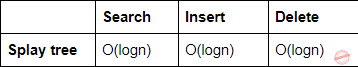Search (or) Find: For the Search (or) Find operation, we perform a normal BST ﬁnd followed by a splay operation on the node found (or the leaf node last encountered, if the key was not found). We can charge the cost of going down the tree to the splay operation. Thus the amortized cost of ﬁnd is O(log n).
Insert/delete: The amortized cost of the splay operation is also O(log n), and thus the amortized cost of insert/delete is O(log n).
 Question 10
Suppose that the splits at every level of Quicksort are in proportion 1-β to β, where 0 < β ≤ 0.5 is a constant. The number of elements in an array is n. The maximum depth is approximately
 A 0.5 β Ig n B 0.5 (1 – β) Ig n C – (Ig n)/(Ig β) D – (Ig n)/Ig (1 – β)
Algorithms       Sorting
 Question 11
The minimum number of nodes in a binary tree of depth d (root is at level 0) is
 A 2d – 1 B 2d + 1 – 1 C d + 1 D d
Data-Structures       Binary-Trees
Question 11 Explanation:
Binary tree is a tree where each node has at most 2 children nodes.
Properties:
→The number of nodes at depth d in a perfect binary tree = 2d
→A perfect binary tree of height h has 2h+1 -1 nodes
→Number of leaf nodes in a perfect binary tree of height h = 2h
→Number of internal nodes in a perfect binary tree of height h = 2h -1
→The minimum number of nodes in a binary tree of height h = h+1
→The maximum number of nodes in a binary tree of height h = 2h+1 -1
 Question 12
The efficient data structure to insert/delete a number in a stored set of numbers is
 A Queue B Linked list C Doubly linked list D Binary tree
Question 12 Explanation:
Doubly linked list is the efficient data structure to insert/delete a number in a stored set of numbers.Question 13
The number of states in a minimal deterministic finite automaton corresponding to the language L = { an | n≥4 } is
 A 3 B 4 C 5 D 6
Theory-of-Computation       DFA
Question 13 Explanation:
L={aaaa, aaaaa, aaaaaa, .........}
The minimal DFA accepting the given language L is given below: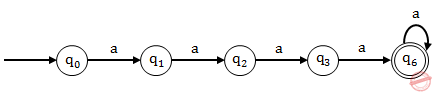Hence the number of states in a minimal deterministic finite automaton corresponding to the language L is 5.
 Question 14
Regular expression for the language L = { w ∈ {0, 1}* | w has no pair of consecutive zeros} is
 A (1 + 010)* B (01 + 10)* C (1 + 010)* (0 + λ) D (1 + 01)* (0 + λ)
Theory-of-Computation       Regular-Expression
Question 14 Explanation:
L={ null, 0, 1, 01, 10, 11, 010, 011, 101, 110, 111, ...........}
Option A is incorrect because it can generate 010010 string which is having a pair of consecutive zeros.
Option B is incorrect because it can generate 1001 string which is having a pair of consecutive zeros.
Option C is incorrect because it can generate 0100 string which is having a pair of consecutive zeros.
Option D is correct because it will generate all the strings mentioned in the given language L
 Question 15
Consider the following two languages :
L1 = {an bl ak | n + l + k>5 }
L2 = {an bl ak | n>5, l >3, k≤ l }
Which of the following is true ?
 A L1 is regular language and L2 is not regular language. B Both L1 and L2 are regular languages. C Both L1 and L2 are not regular languages. D L1 is not regular language and L2 is regular language
Theory-of-Computation       Regular-Language
Question 15 Explanation:
→ L1 is regular → L2 is not a regular language because of "k≤ l" condition, this condition needs the machine which can remember number of b's in the language so that number of a's following "b" can be compared. Since Finite automata don't have any memory unit to remember the previous inputs, So it can't accept the language L2. Hence L2 is not a regular language.
 Question 16
LL grammar for the language L = {an bm cn+m | m≥0, n≥0} is
 A S → aSc | S1 ; S1 → bS1c | λ B S → aSc | S1| λ ; S1 → bS1c C S → aSc | S1| λ ; S1 → bS1c| λ D S → aSc | λ ; S1 → bS1c| λ|
Theory-of-Computation       Languages-and-Grammars
Question 16 Explanation:
L1 is the language when n=0, m=0.
L1={λ}
L2 is the language when only n=0
L2={bc, bbcc, bbbccc,......}
L3 is the language when only m=0
L3={ ac, aacc, aaaccc, ........}
L4 is the language when n≠0 and m≠0
L4= {abcc, aabbcccc, aaabbbcccccc,...............}
L= {L1 U L2 U L3 U L4}
L= { λ, bc, bbcc, bbbccc,........, ac, aacc, aaaccc,.........., abcc, aabbcccc,aaabbbcccccc,.........}
Option A is incorrect because it does not have any stoping point for condition when m=0 i.e it can't generate strings { ac, aacc, aaaccc, ........}
Option B is incorrect because it does not have any stopping point for condition when n=0 i.e it can't generate strings {bc, bbcc, bbbccc,......}
Option C is correct because it can generate all the strings generated by the language L.
Option D is incorrect because state S1 is unreachable in it. And because of this strings {bc, bbcc, bbbccc,......{abcc, aabbcccc, aaabbbcccccc,...............} can't be generated.
 Question 17
Assume the statements S1 and S2 given as : S1 : Given a context free grammar G, there exists an algorithm for determining whether L(G) is infinite. S2 : There exists an algorithm to determine whether two context free grammars generate the same language. Which of the following is true ?
 A S1 is correct and S2 is not correct. B Both S1 and S2 are correct. C Both S1 and S2 are not correct. D S1 is not correct and S2 is correct.
Theory-of-Computation       Context-Free-Grammar
Question 17 Explanation:
Statement S1 is correct because finiteness problem of CFG is decidable
. Statement S2 is incorrect because equality problem (L1 = L2; where L1 and L2 are CFL) of CFG is undecidable .
 Question 18
The number of eight-bit strings beginning with either 111 or 101 is______.
 A 64 B 128 C 265 D None of the above
Engineering-Mathematics       Combinatorics
Question 18 Explanation:
Given data,
-- Total number of bits=8
-- Beginning with either 111 or 101
-- Total number of strings=?
Step-1: First consider 111 as beginning numbers. It look like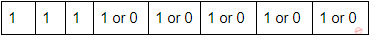Possibility to get combinations are 25 =32 Step-2: Second consider 101 as beginning numbers. It look likePossibility to get combinations are 25 =32 Step-3: Total number of strings are 32+32
=64
 Question 19
Find the number of ways to paint 12 offices so that 3 of them will be green, 2 of them pink, 2 of them yellow and the rest ones white.
 A 55,440 B 1,66,320 C 4.790E+08 D 39,91,680
Engineering-Mathematics       Combinatorics
Question 19 Explanation:
Given data,
-- Total number of offices=12
-- Colours are Green=3, Pink=2, Yellow=2 and White=5
white= 12-7
= 5
-- Total number of ways to paint 12 offices=?
Step-1: Total number of offices are 12 then we get maximum 12!
12!=479001600
Step-2: Green=3, Pink=2, Yellow=2 and White=5
maximum possibilities of individual colours are
= 3! * 2! * 2! * 5!
= 2880
Step-3: Total number of ways to paint 12 offices= (12! / (3! * 2! * 2! * 5!))
= 479001600 / 2880
= 166320
 Question 20
Consider the following statements :
(i) A graph in which there is a unique path between every pair of vertices is a tree.
(ii) A connected graph with e = v – 1 is a tree.
(iii) A graph with e = v – 1 that has no circuit is a tree.
Which of the above statements is/are true ?
 A (i) & (iii) B (ii) & (iii) C (i) & (ii) D All of the above
Engineering-Mathematics       Graph-Theory
Question 20 Explanation:

Tree properties:

→A graph in which there is a unique path between every pair of vertices is a tree.
→A connected graph with e = v – 1 is a tree.
→A graph with e = v – 1 that has no circuit is a tree.
 Question 21
Consider the In-order and Post-order traversals of a tree as given below :
In-order : j e n k o p b f a c l g m d h I
Post-order : j n o p k e f b c l m g h i d a
The Pre-order traversal of the tree shall be
 A a b f e j k n o p c d g l m h i B a b c d e f j k n o p g l m h i C a b e j k n o p f c d g l m h i D j e n o p k f b c l m g h i d a E None of the above
Data-Structures       Binary-Trees
Question 21 Explanation:
Step-1: Post order traversal is Left, Right and Root according to post order we are dividing into in-order list.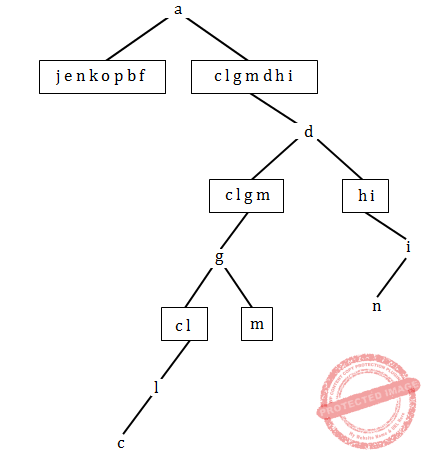Step-2:Step-3: Final tree isStep-4: Preorder traversal is Root,left and right
preorder= a b e j k n p o f d g l c m i h
Note: Given options are wrong. Excluded for evaluation.
 Question 22
A simple graph G with n-vertices is connected if the graph has
 A (n – 1) (n – 2)/2 edges B more than (n – 1) (n – 2)/2 edges C less than (n – 1) (n – 2)/2 edges D Σki=1 C(ni, 2) edges
Engineering-Mathematics       Graph-Theory
Question 22 Explanation:
The simple graph won’t have parallel edges and self loops.
Simple graph properties:
→If a graph is connected then e≥n-1.
→If a graph has more than e>((n-1)(n-2)) / 2 then it is connected.
→The n vertex graph with the maximal number of edges that is still disconnected is a Kn-1
→A complete graph Kn-1 with n-1 vertices ((n-1)(n-2)) / 2 edges.
→Adding any possible edge must connect the graph, so the minimum number of edges needed to guarantee connectivity for an ‘n’ vertex graph is (((n−1)(n−2)) / 2)+1
 Question 23
Which one of the following set of gates is best suited for ‘parity’ checking and ‘parity’ generation ?
 A AND, OR, NOT B NAND, NOR C EX-OR, EX-NOR D None of the above
Digital-Logic-Design       Logic-Gates
Question 23 Explanation:
‘parity’ checking using EX-OR and ‘parity’ generation using EX-NOR
Example:
Even parity Check using EX-OR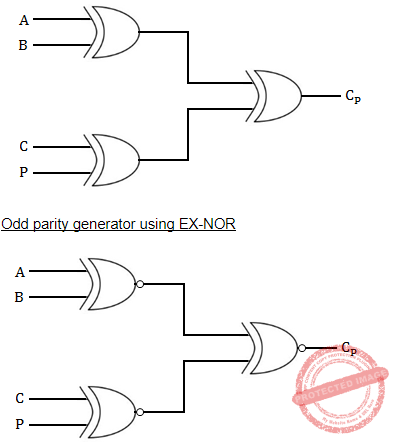Question 24
The quantification ∃!x P(x) denotes the proposition “There exists a unique x such that P(x) is true”,express the quantification using universal and existential quantification's and logical operators :
 A ∃x P(x) ∨ ∀x∀y ((P(x) ∨ P(y)) → x = y) B ∀x P(x) ∧ ∀x∀y ((P(x) ∨ P(y) → x = y) C ∃x P(x) ∧ ∀x∀y ((P(x) ∧ P(y)) → x = y) D ∃x P(x) ∧ ∃x∃y ((P(x) ∨ P(y)) → x = y)
Engineering-Mathematics       Propositional-Logic
Question 24 Explanation:
According to the given data, the possible solution is ∃x P(x) ∧ ∀x∀y ((P(x) ∧ P(y)) → x = y)
 Question 25
If F and G are Boolean functions of degree n. Then, which of the following is true ?
 A F ≤ F + G and F G ≤ F B G ≤ F + G and F G ≥ G C F ≥ F + G and F G ≤ F D G ≥ F + G and F G ≤ F
Digital-Logic-Design       Boolean-Function
Question 25 Explanation:
Given data,
-- F and G are boolean functions of degree n.
Step-1: Let n=4
Using n=22^n formula, we can find number of boolean functions
= 22^4
= 256
Stp-2: First consider F4→ F & G4→ G
F having 256 boolean functions and G having 256 boolean functions.
Option-A: F + G= 512 boolean functions and F*G= 14336 boolean functions.
F ≤ F + G and F G ≤ F FALSE because F G ≤ F is wrong
Option-B: G ≤ F + G and F G ≥ G TRUE
F + G= 512 boolean functions and F*G= 14336 boolean functions.
Option-C: F ≥ F + G and F G ≤ F FALSE because both are wrong
Option-D: G ≥ F + G and F G ≤ F FALSE because F G ≤ F is wrong.
 Question 26
Match the following identities/laws to their corresponding name :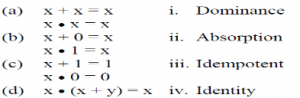A a-iii, b-iv, c-i, d-ii B a-iv, b-iii, c-i, d-ii C a-iv, b-iii, c-ii, d-i D a-iii, b-iv, c-ii, d-i
Question 26 Explanation:
Idempotent laws: x+x=x and x.x=x
Identity laws: x+0=x and x.1=x
Dominance laws: x+1=1 and x.0=0
Absorption laws: x.(x+y)=x
 Question 27
In which one of the following, continuous process improvement is done ?
 A ISO9001 B RMMM C CMM D None of the above
Question 27 Explanation:
CMM stands for Capability Maturity Model is a process model which specifies the process improvement approach in software development.
CMM levels:
→Initial
→Repeatable
→Defined
→Managed
→Optimizing
 Question 28
The ______ of a program or computing system is the structure or structures of the system, which comprise software components, the externally visible properties of these components, and the relationship among them.
 A E-R diagram B Data flow diagram C Software architecture D Software design
Software-Engineering       Software-requirements
Question 28 Explanation:
The software architecture of a program or computing system is the structure or structures of the system, which comprise software components, the externally visible properties of these components, and the relationship among them.
 Question 29
Working software is not available until late in the process in
 A Waterfall model B Prototyping model C Incremental model D Evolutionary Development model
Software-Engineering       Waterfall-model
Question 29 Explanation:
Waterfall Model: We can not go back in previous project phase as soon as as we proceed to next phase ,So inflexible
→Waterfall model is simple and easy to understand.
→It works as reference model for others.
→Real projects cannot be sequential
→Initially all requirements should known i.e requirements are frozen. It may be suited for static projects.
→Error omission too costly
→Maintenance is too costly(We have to redesign the whole project from scratch)
→Customer must have patience
→It is based on Big-Bang approach
 Question 30
Equivalence partitioning is a ______ testing method that divides the input domain of a program into classes of data from which test cases can be derived.
 A White box B Black box C Regression D Smoke
Software-Engineering       Software-testing
Question 30 Explanation:
Equivalence partitioning is a black box testing method that divides the input domain of a program into classes of data from which test cases can be derived.
Black box testing methods are
→Graph-Based Testing Methods
→Equivalence Partitioning
→Boundary Value Analysis
→Orthogonal Array Testing
 Question 31
Consider the following characteristics :
(i) Correct and unambiguous
(ii) Complete and consistent
(iii) Ranked for importance and/or stability and verifiable
(iv) Modifiable and Traceable
Which of the following is true for a good SRS ?
 A (i), (ii) and (iii) B (i), (iii) and (iv) C (ii), (iii) and (iv) D (i), (ii), (iii) and (iv)
Software-Engineering       Software-requirements
Question 31 Explanation:
A good software requirement specification(SRS) characteristics are
→Correct and unambiguous
→Complete and consistent
→Ranked for importance and/or stability and verifiable
→Modifiable and Traceable
 Question 32
Linked Lists are not suitable for _____.
 A Binary Search B Polynomial Manipulation C Insertion D Radix Sort
Question 32 Explanation:
Linked Lists are not suitable for binary search.
Note: We can also implement binary search using linked list but it will give time complexity is O(n) instead of O(logn).
 Question 33
What is the size of the following Union ? Assume that the size of int = 2, size of float = 4, size of char = 1

union tag {

int a;

float b;

char c;

};

 A 2 B 4 C 1 D 7
Programming       Structure
Question 33 Explanation:
Union: The compiler allocates the memory by considering the size of the largest memory. So, size of union is equal to the size of largest member
union tag
{
int a; /* It takes only 2 bytes */
float b; /* It takes only 4 bytes */
char c; /* It takes only 1 bytes */
};
As per the definition of union will take 4 bytes of memory. Because float having highest size among all.
 Question 34
What is the output of the following program segment ?

sum(n)

{

if ( n < 1 ) return n;

else return (n + sum(n–1));

}

main()

{

printf(“%d”, sum(5));

}

 A 10 B 16 C 15 D 14
Operating-Systems       System-Calls
Question 34 Explanation:
Here, n=5
Given condition is
if ( n < 1 ) return n;
else return (n + sum(n–1));
Iteration-1: if(5<1) FALSE
5+sum(4)
Iteration-2: if(4<1) FALSE
4+sum(3)
Iteration-3: if(3<1) FALSE
3+sum(2)
Iteration-4: if(2<1) FALSE
2+sum(1)
Iteration-5: if(1<1) TRUE
return 1
Total cost= 5+4+3+2+1
= 15
 Question 35
Assume that x and y are non-zero positive integers. What does the following program segment perform ? while (x!=0) { if (x>y)     x = x-y;     else      y=y-x; printf(“%d”,x); }
 A Computes LCM of two numbers B Computes GCD of two numbers C Divides large number with small number D Subtracts smaller number from large number
Programming       Control-Statement
Question 35 Explanation:
Let X=3 and Y=5
1st pass : X=3 and Y=2
2nd pass : X=1 and Y=2
3rd pass : X=1 and Y=1
Write(X), which writes 1.
Which is nothing but GCD of 3 & 5.
 Question 36
Consider the following program segment :

d=0;

for(i=1; i<31, ++i)

for(j=1; j<31, ++j)

for(k=1; k<31, ++k)

if ((i+j+k)%3)= = 0);

d = d + 1;

printf(“%d”, d);

The output will be

 A 9000 B 3000 C 90 D 2700
Programming       Control-Statement
Question 36 Explanation:
Step1: Each for loop will execute 30 times, then total number of iterations are 30*30*30=27000,
But value “d” is incremented only factors of 3 only.
Step2: For the values i=1, j=1 then
k=1,4,7,10,13,16,19,22,25 and 28(10 times)
i=1 and j=2 then
k=3,6,9,12,15,18,21,24,27,and 30(10 times)
i=1 and j=3 then
K=2,5,8,11,14,17,20,23,26,29(10 times)
Then for i=1 value there will be 30*10 =300 times.
Finally for all “i” values from 1 to 30 is
= 300*30
= 9000
 Question 37
Usage of Preemption and Transaction Rollback prevents ______.
 A Unauthorised usage of data file B Deadlock situation C Data manipulation D File preemption
Database-Management-System       Transactions
Question 37 Explanation:
Usage of Preemption and Transaction Rollback prevents deadlock.
 Question 38
The _____ language was originally designed as the Transformation Language for Style Sheet facility.
 A XSTL B XML C XQuery D XPath
Web-Technologies       XML
Question 38 Explanation:
XSLT (eXtensible Stylesheet Language Transformations) is the recommended style sheet language for XML.
XSLT is far more sophisticated than CSS. With XSLT you can add/remove elements and attributes to or from the output file. You can also rearrange and sort elements, perform tests and make decisions about which elements to hide and display, and a lot more.
XSLT uses XPath to find information in an XML document.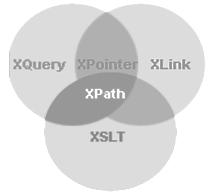Question 39
Views are useful for _____ unwanted information, and for collecting together information from more than one relation into a single view.
 A Hiding B Deleting C Highlighting D All of the above
Database-Management-System       SQL
Question 39 Explanation:
→ Views are useful for hides unwanted information, and for collecting together information from more than one relation into a single view.
→ A view is the result set of a stored query on the data, which the database users can query just as they would in a persistent database collection object.
Views advantages over tables:
→Views can represent a subset of the data contained in a table. Consequently, a view can limit the degree of exposure of the underlying tables to the outer world: a given user may have permission to query the view, while denied access to the rest of the base table. →Views can join and simplify multiple tables into a single virtual table.
→Views can act as aggregated tables, where the database engine aggregates data (sum, average, etc.) and presents the calculated results as part of the data.
→Views can hide the complexity of data. For example, a view could appear as Sales2000 or Sales2001, transparently partitioning the actual underlying table.
→Views take very little space to store; the database contains only the definition of a view, not a copy of all the data that it presents.
→Depending on the SQL engine used, views can provide extra security.
 Question 40
The decision tree classifier is a widely used technique for ______.
 A Classification B Association C Partition D Clustering
Question 40 Explanation:
The decision tree classifier is a widely used technique for classification.
 Question 41
Cross_tab displays permit users to view ______ of multidimensional data at a time.
 A One dimension B Two dimensions C Three dimensions D Multidimensions
Question 41 Explanation:
Cross_tab displays permit users to view two dimensions of multidimensional data at a time.
 Question 42
A method to provide secure transmission of email is called ____.
 A TLS B SA C IPSec D PGP
Question 42 Explanation:
A method to provide secure transmission of email is called Pretty Good Privacy(PGP). PGP is used for signing, encrypting, and decrypting texts, e-mails, files, directories, and whole disk partitions and to increase the security of e-mail communications.
 Question 43
Thomas-write rule is ______.
 A Two phase locking protocol B Timestamp ordering protocol C One phase locking protocol D Sliding window proto
Database-Management-System       Transactions
Question 43 Explanation:
→ The Thomas write rule is a rule in timestamp-based concurrency control. It can be summarized as ignore outdated writes.
→ The Thomas write rule is applied in situations where a predefined logical order is assigned to transactions when they start.
 Question 44
Match the following :A a-iii, b-i, c-ii, d-iv B a-iv, b-i, c-iii, d-ii C a-iv, b-iii, c-i, d-ii D a-iii, b-iii, c-ii, d-i
Operating-Systems       Process-Scheduling
Question 44 Explanation:
Ready→ Running: The process is dispatched.
Blocked→ Ready: Request made by the process is satisfied or an event for which it was waiting occurs.
Running→ Blocked: Process wishes to wait for some action by another process.
Running→ Ready: The process is preempted.
 Question 45
The hit ratio of a Translation Lookaside Buffer (TLAB) is 80%. It takes 20 nanoseconds (ns) to search TLAB and 100 ns to access main memory. The effective memory access time is ______.
 A 36 ns B 140 ns C 122 ns D 40 ns
Operating-Systems       Memory-Management
Question 45 Explanation:
Given data,
-- hit ratio=80% it is equivalent to 0.8
-- search time=20 ns
-- access memory=100 ns
-- miss ratio= 1-Hit ratio
= 1-0.8
= 0.2
-- Effective access memory=?
Step-1: Effective access memory= Hit ratio*(search time+access memory) + Miss ratio*(search time+2*access memory)
= 0.8*(120)+0.2*(220) ns
= 140 ns
 Question 46
Consider the input/output (I/O) requests made at different instants of time directed at a hypothetical disk having 200 tracks as given in the following table :Assume that : Current head position is at track no. 65 Direction of last movement is towards higher numbered tracks Current clock time is 160 milliseconds Head movement time per track is 1 millisecond. “look” is a variant of “SCAN” disk arm scheduling algorithm. In this algorithm, if no more I/O requests are left in current direction, the disk head reverses its direction. The seek times in Shortest Seek First (SSF) and “look” disk-arm scheduling algorithms respectively are :
 A 144 and 123 milliseconds B 143 and 123 milliseconds C 149 and 124 milliseconds D 256 and 186 milliseconds
Operating-Systems       Disk-scheduling
Question 46 Explanation:
Given data
Current head position is at track no. 65
The direction of last movement is towards higher numbered tracks
Current clock time is 160 milliseconds
Head movement time per track is 1 millisecond.According to clock time, the serial no 1,2,3 and 4 tracks are ready to process.
SSTF( Shortest Seek time First )
Current head position is 65, according to SSTF next track is 75 but it will arrive at the time of 175, then it will process 85 first, then track 75 later 100 from there it will process 40 and then 12
so the requests are 65 → 85→ 75→ 100→ 40→ 12
= (85-65)+(85-75)+(100-75)+(100-40)+(40-12)
= 20+10+25+60+28
= 143 ms
Look method:
The requests are processed in the following way
Direction of last movement is towards higher number of tracks.
65→ 85→ 100→ 75→ 40→ 12
=(85-65)+(100-85)+(100-75)+(75-40)+(40-12)
=20+15+25+35+28
=123ms
Given data
Current head position is at track no. 65
The direction of last movement is towards higher numbered tracks
Current clock time is 160 milliseconds
Head movement time per track is 1 millisecond.According to clock time, the serial no 1,2,3 and 4 tracks are ready to process.
SSTF( Shortest Seek time First )
Current head position is 65, according to SSTF next track is 75 but it will arrive at the time of 175, then it will process 85 first, then track 75 later 100 from there it will process 40 and then 12
so the requests are 65 → 85→ 75→ 100→ 40→ 12
= (85-65)+(85-75)+(100-75)+(100-40)+(40-12)
= 20+10+25+60+28
= 143 ms
Look method:
The requests are processed in the following way
Direction of last movement is towards higher number of tracks.
65→ 85→ 100→ 75→ 40→ 12
=(85-65)+(100-85)+(100-75)+(75-40)+(40-12)
=20+15+25+35+28
=123ms
 Question 47
Assume that an implementation of Unix operating system uses i-nodes to keep track of data blocks allocated to a file. It supports 12 direct block addresses, one indirect block address and one double indirect block address. The file system has 256 bytes block size and 2 bytes for disk block address. The maximum possible size of a file in this system is
 A 16 MB B 16 KB C 70 KB D 71 KB E None of the Above
Operating-Systems       File system-I/O-protection
Question 47 Explanation:
Block size = 28 KB
Size of one address = 2 Bytes
No. of addresses a block can point/contain =28 /2=27
Max. file size =(12+27+(27 * 27))28
=222 GB
=4 MB Note: Wrong Question
 Question 48
Which of the following set of Unix commands will always display “WELCOME” ?
 A export title=WELCOME; Echo \$title B title = WELCOME; export \$ title ; sh –c “echo \$title” C title = WELCOME; export title ; sh –c “echo \$title” D title = WELCOME; echo \$title
Question 48 Explanation:
title = WELCOME;
export title ; /* The export command marks an environment variable to be exported with any newly forked child processes and thus it allows a child process to inherit all marked variables.*/
sh –c “echo \$title”; /* sh calls the program sh as interpreter and the -c flag means execute the following command as interpreted by this program. */
echo→ will display the content of a variable.
 Question 49
What type of logic circuit is represented by the figure shown below ?A XOR B XNOR C XAND D XNAND
Digital-Logic-Design       Logic-Gates
Question 49 Explanation: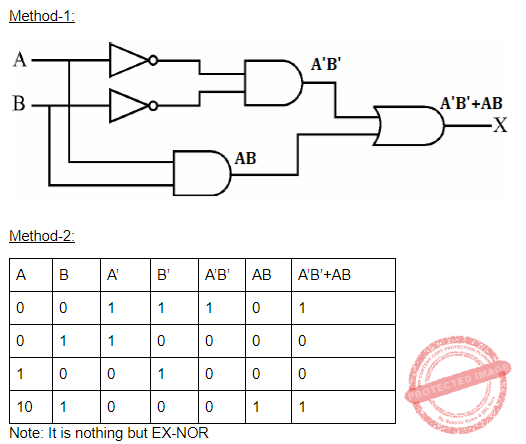Question 50
The speed up of a pipeline processing over an equivalent non-pipeline processing is defined by the ratio :   Where n → no. of tasks t<sub>n</sub>→ time of completion of each task k → no. of segments of pipeline t<sub>p</sub> → clock cycle time S → speed up ratio
 A S =n tn/(k + n – 1)tp B S =n tn/(k + n + 1)tp C S =n tn/(k – n + 1)tp D S =(k + n – 1)tp/n tn
Computer-Organization       Pipelining
Question 50 Explanation:
Without pipeline one task needs tn time. So, n tasks need n*tn time. T without pipeline = n*tn With pipeline: First task needs k cycles to finish. So time for 1st task will be k*tp Each of the other n-1 tasks need tp time only to finish. So, T pipeline = (k+n-1)*tp Speed up = T without pipeline / T pipeline = n*tn / (k+n-1)*tp
There are 50 questions to complete.
PHP Code Snippets Powered By : XYZScripts.com